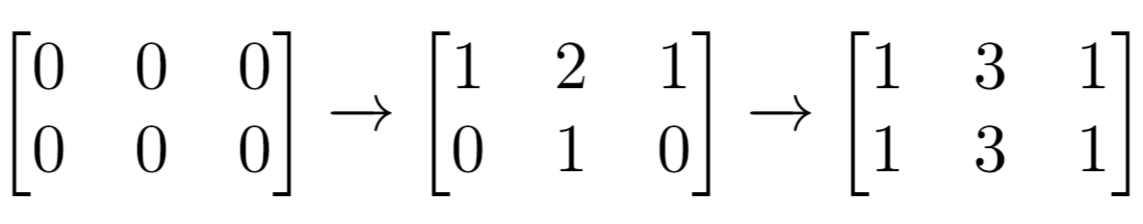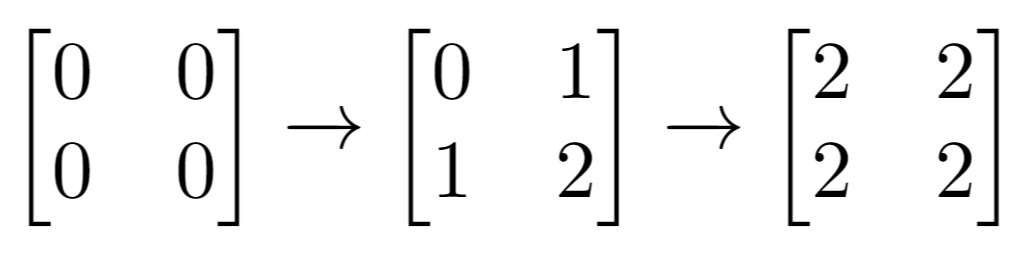1252. Cells with Odd Values in a Matrix

There is an `m x n` matrix that is initialized to all `0`'s. There is also a 2D array `indices` where each `indices[i] = [ri, ci]` represents a 0-indexed location to perform some increment operations on the matrix.

For each location `indices[i]`, do both of the following:

1. Increment all the cells on row `ri`.
2. Increment all the cells on column `ci`.

Given `m`, `n`, and `indices`, return the number of odd-valued cells in the matrix after applying the increment to all locations in `indices`.

Example 1:```Input: m = 2, n = 3, indices = [[0,1],[1,1]]
Output: 6
Explanation: Initial matrix = [[0,0,0],[0,0,0]].
After applying first increment it becomes [[1,2,1],[0,1,0]].
The final matrix is [[1,3,1],[1,3,1]], which contains 6 odd numbers.
```

Example 2:```Input: m = 2, n = 2, indices = [[1,1],[0,0]]
Output: 0
Explanation: Final matrix = [[2,2],[2,2]]. There are no odd numbers in the final matrix.
```

Constraints:

• `1 <= m, n <= 50`
• `1 <= indices.length <= 100`
• `0 <= ri < m`
• `0 <= ci < n`

Follow up: Could you solve this in `O(n + m + indices.length)` time with only `O(n + m)` extra space?

1252. Cells with Odd Values in a Matrix
``````struct Solution;

impl Solution {
fn odd_cells(n: i32, m: i32, indices: Vec<Vec<i32>>) -> i32 {
let n = n as usize;
let m = m as usize;
let mut a = vec![vec![0; m]; n];
let mut res = 0;
for p in indices {
let r = p as usize;
let c = p as usize;
for j in 0..m {
a[r][j] += 1;
if a[r][j] % 2 == 1 {
res += 1;
} else {
res -= 1;
}
}
for i in 0..n {
a[i][c] += 1;
if a[i][c] % 2 == 1 {
res += 1;
} else {
res -= 1;
}
}
}
res
}
}

#[test]
fn test() {
let n = 3;
let m = 3;
let indices: Vec<Vec<i32>> = vec_vec_i32![[0, 1], [1, 1]];
assert_eq!(Solution::odd_cells(n, m, indices), 6);
let n = 2;
let m = 2;
let indices: Vec<Vec<i32>> = vec_vec_i32![[1, 1], [0, 0]];
assert_eq!(Solution::odd_cells(n, m, indices), 0);
}
``````